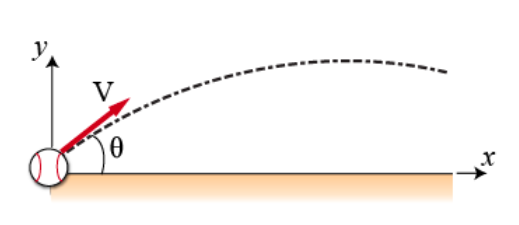# Problem: During a baseball game, a baseball is struck at ground level by a batter. The ball leaves the baseball bat with an initial velocity v0 = 25 m/s at an angle θ = 15° above horizontal. Let the origin of the Cartesian coordinate system be the ball's position the instant it leaves the bat. Air resistance may be ignored throughout this problem.Part A. Create an expression in terms of v0, θ, and g for the time tmax it takes the ball to travel to its maximum vertical height.Part B. Calculate the horizonal distance xmax in meters the ball has traveled when it returns to ground level.

###### FREE Expert Solution

2D vectors Components:

Range:

$\overline{){\mathbf{R}}{\mathbf{=}}\frac{{{\mathbf{v}}_{\mathbf{0}}}^{\mathbf{2}}\mathbf{s}\mathbf{i}\mathbf{n}\mathbf{2}\mathbf{\theta }}{\mathbf{g}}}$

Part A

Time taken to travel to maximum height, tmax is found by:

First, consider the kinematic equation vf = v0 + at

At maximum height, vfy = 0###### Problem Details

During a baseball game, a baseball is struck at ground level by a batter. The ball leaves the baseball bat with an initial velocity v0 = 25 m/s at an angle θ = 15° above horizontal. Let the origin of the Cartesian coordinate system be the ball's position the instant it leaves the bat. Air resistance may be ignored throughout this problem.Part A. Create an expression in terms of v0, θ, and g for the time tmax it takes the ball to travel to its maximum vertical height.

Part B. Calculate the horizonal distance xmax in meters the ball has traveled when it returns to ground level.